Related Articles

# Count non zero values in each column of R dataframe

• Last Updated : 05 Apr, 2021

In this article, we are going to count the number of non-zero data entries in the data using R Programming Language.

To check the number of non-zero data entries in the data first we have to put that data in the data frame by using:

```data <- data.frame(x1 = c(1,2,0,100,0,3,10),
x2 = c(5,0,1,8,10,0,0),
x3 = 0)

print(data)```

Output: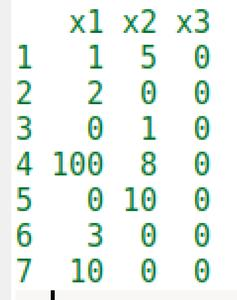Now we have the data in the data frame. So, to count the number of non-zeroes entries in each column we use colSums() function. This function is used as:

`colSums( data != 0)`

Output: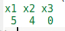As you can clearly see that there are 3 columns in the data frame and Col1 has 5 nonzeroes entries (1,2,100,3,10) and Col2 has 4 non-zeroes entries (5,1,8,10) and Col3 has 0 non-zeroes entries.

Example 1: Here we are going to create a dataframe and then count the non-zero values in each column.

## R

 `# Create example data frame``data <- ``data.frame``(x1 = ``c``(1,2,0,100,0,3,10),   ``                   ``x2 = ``c``(5,0,1,8,10,0,0),``                   ``x3 = 0)`` ` `#  print the dataframe``print``(data) `` ` `# check for every non zero entry using "data!=0" ``# and sum the number of entries using colSums()``colSums``(data != 0)`

Output: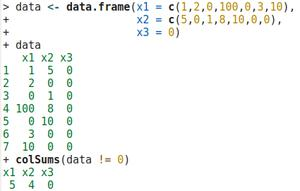Example 2: In this example, we are using iris3 dataset is used.

## R

 `# put the iris3 data in dataframe``data <- ``data.frame``(iris3)`` ` `# check the dimensions of dataframe``dim``(data)`` ` `# check for every non zero entry using "data!=0" ``# and sum the number of entries using colSums()``colSums``(data != 0)`

Output: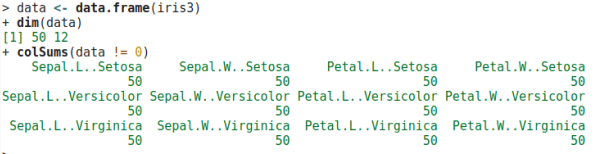Example 3: In this example, the state.x77 dataset is used.

## R

 `# put the state.x77 data in dataframe``data <- ``data.frame``(state.x77)`` ` `# check the dimensions of dataframe``dim``(data)  `` ` `# check for every non zero entry using "data!=0" ``# and sum the number of entries using colSums()``colSums``(data != 0)`

Output: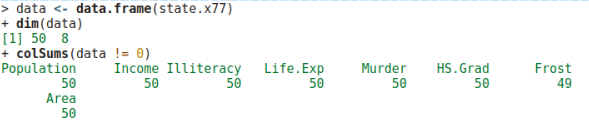Example 4: In this example, the USArrest dataset is used.

## R

 `# put the USArrest data in dataframe``data <- ``data.frame``(USArrest)`` ` `# check the dimensions of dataframe``dim``(data)  `` ` `# check for every non zero entry using "data!=0" ``# and sum the number of entries using colSums()``colSums``(data != 0)`

Output: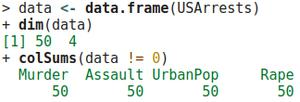My Personal Notes arrow_drop_up